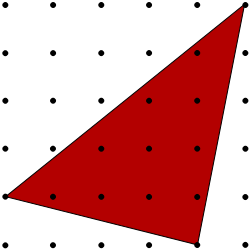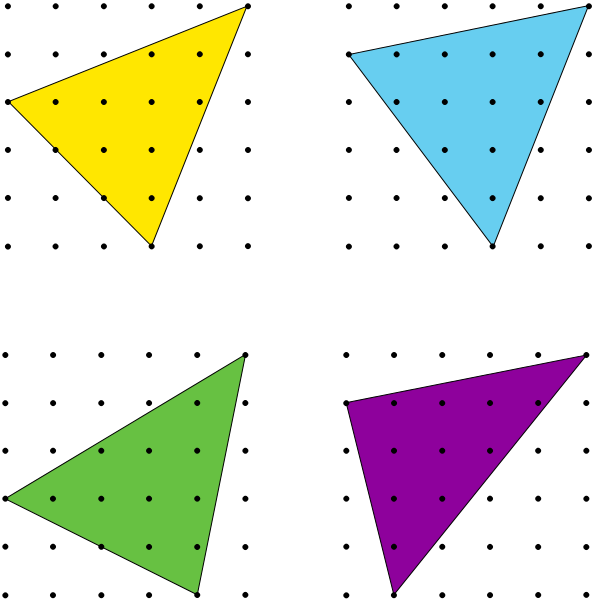#### You may also like### Kissing Triangles

Determine the total shaded area of the 'kissing triangles'.### Isosceles

Prove that a triangle with sides of length 5, 5 and 6 has the same area as a triangle with sides of length 5, 5 and 8. Find other pairs of non-congruent isosceles triangles which have equal areas.Four rods, two of length a and two of length b, are linked to form a kite. The linkage is moveable so that the angles change. What is the maximum area of the kite?

# Triangles in a Square

##### Age 11 to 14Challenge Level

You may wish to print a dotty grid to work on this problem.Here is a triangle drawn on a $5$ by $5$ dotty grid by joining the top-right-hand dot to a dot on the left hand side of the grid, and a dot on the bottom of the grid.

Here are some more triangles drawn in the same way.
Which has the largest area?Now, think about the different triangles that can be formed with a vertex at $(5,5)$, a vertex on the left hand side and a vertex on the bottom of the grid.

What is the smallest area such a triangle can have?
Can you find a general expression for the area of a triangle on this grid if its vertices have co-ordinates $(5,5)$, $(x,0)$ and $(0,y)$?
What can you say about the areas of triangles drawn on a $6$ by $6$ grid? Or a $7$ by $7$ grid? Or a $100$ by $100$ grid...?